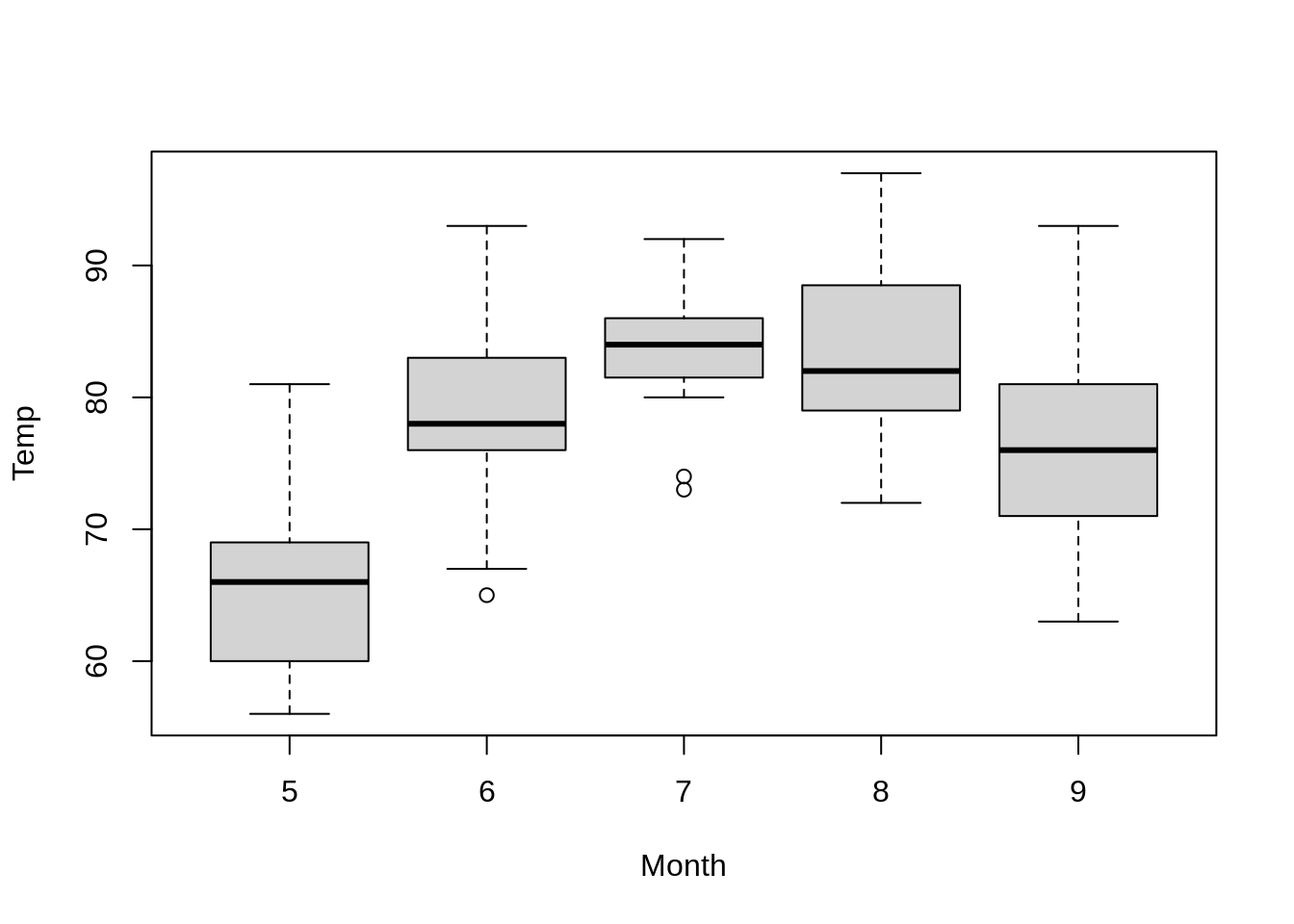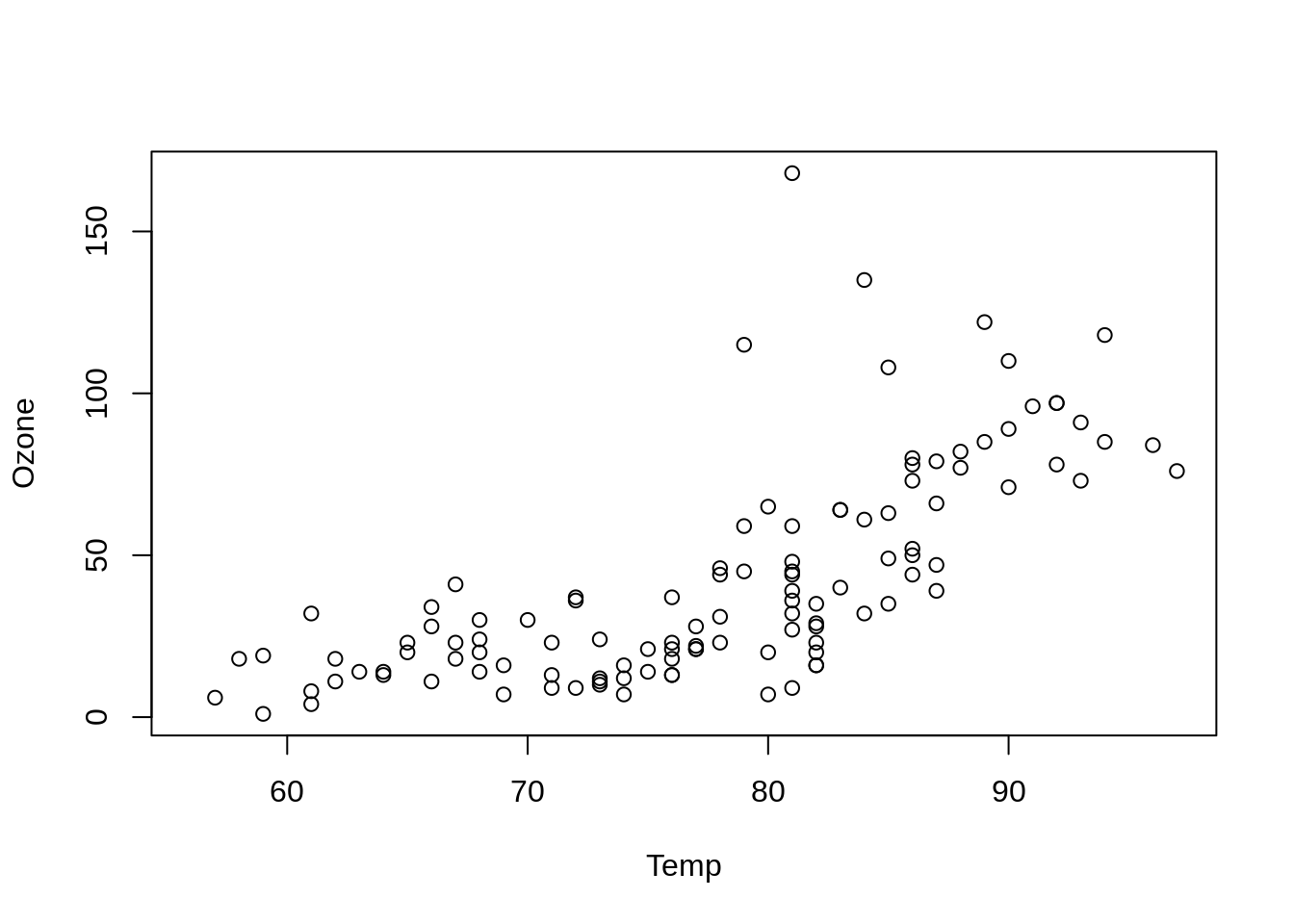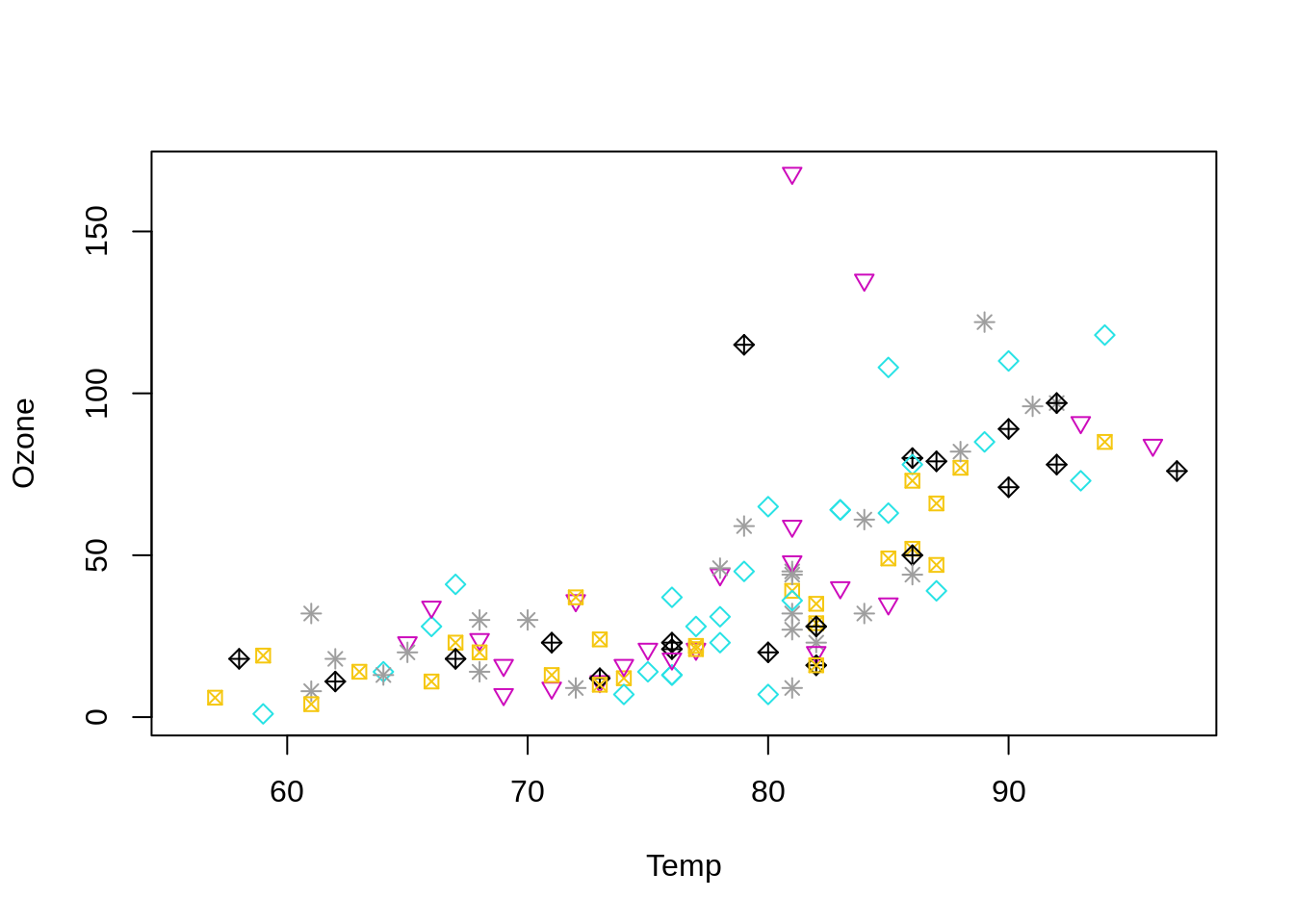## Air quality

``````with(airquality, boxplot(Temp ~ Month))
````````````with(airquality, plot(Ozone ~ Temp))
````````````mlev <- levels(with(airquality, as.factor(Month)))
with(airquality, plot(Ozone ~ Temp,
pch = as.numeric(mlev),
col = mlev))
``````Posted on:
December 30, 2020
Length:
1 minute read, 24 words